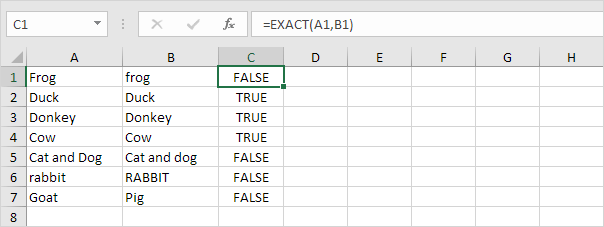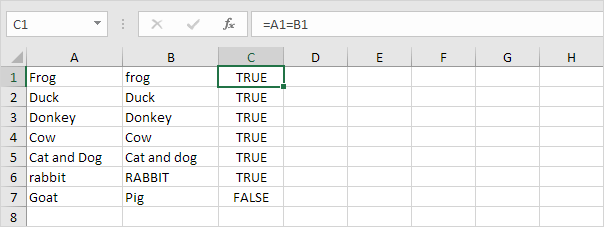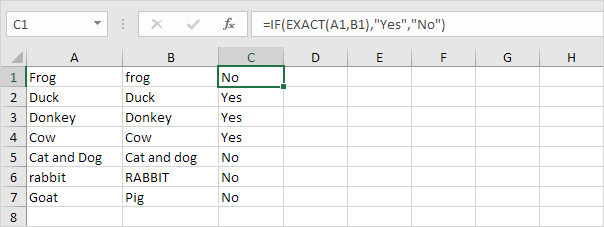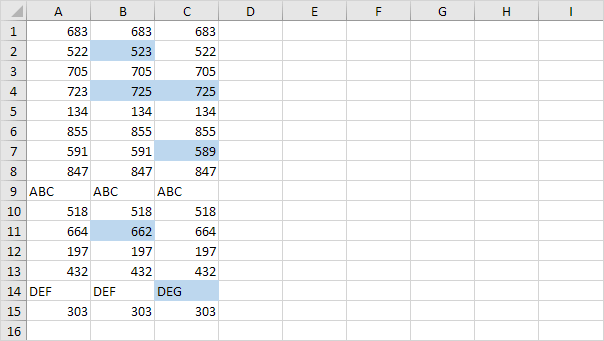# Compare Text

This example shows two ways to compare text strings in Excel. One is case-sensitive and one is case-insensitive.

1. Use the EXACT function (case-sensitive).Explanation: the string "Frog" in cell A1 and the string "frog" in cell B1 are not exactly equal to each other (first letter in uppercase and first letter in lowercase).

2. Use the formula =A1=B1 (case-insensitive).Explanation: this formula ignores lowercase and uppercase differences. As a result, the first six formulas return TRUE.

3. Add the IF function to replace TRUE and FALSE with a word or message. The formula below uses the EXACT function (see step 1).4. Do you want to compare two or more columns by highlighting the differences in each row? Visit our page about Row Differences.